# Unit 4 Test Review Chapter 6 Packet Lessons

• Slides: 32
Download presentationUnit 4 Test Review Chapter 6 Packet Lessons 1 -8 Chapter 8 Book Lesson 6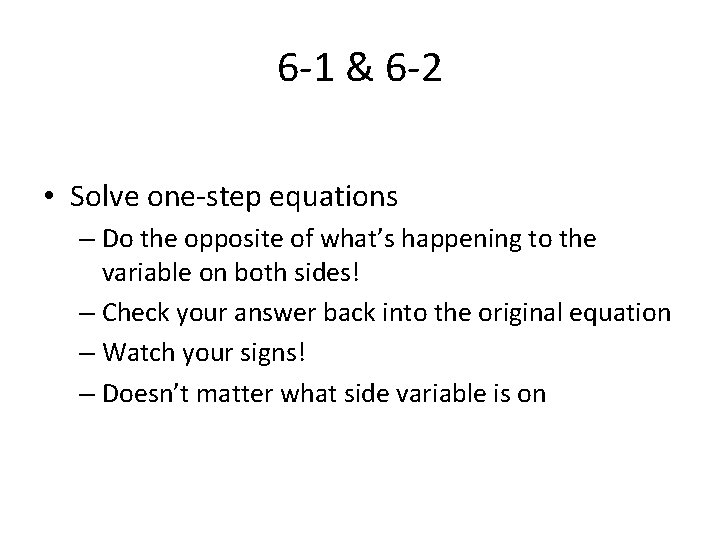6 -1 & 6 -2 • Solve one-step equations – Do the opposite of what’s happening to the variable on both sides! – Check your answer back into the original equation – Watch your signs! – Doesn’t matter what side variable is on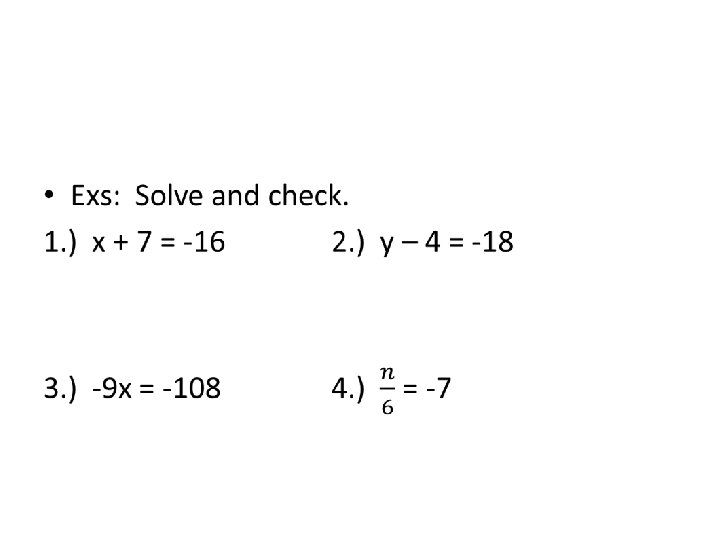• Ex: • El Rodeo School has 837 students. El Rodeo School has 78 more students than Hawthorne. How many students are there at Hawthorne School? Write an equation and then solve.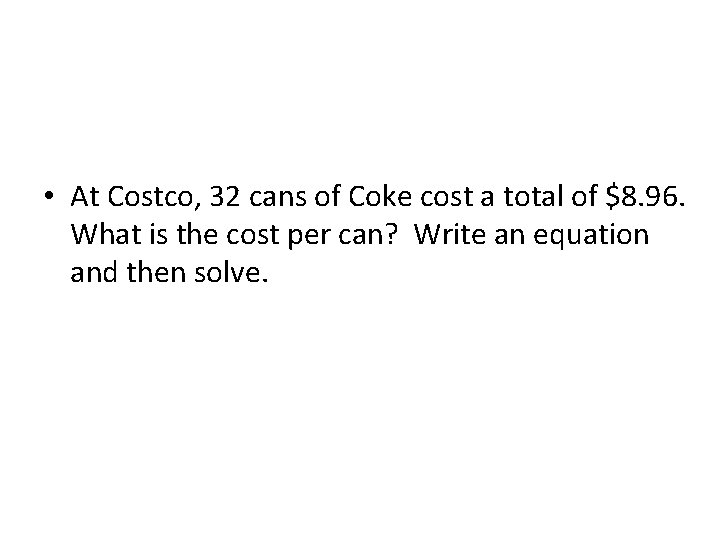• At Costco, 32 cans of Coke cost a total of \$8. 96. What is the cost per can? Write an equation and then solve.6 -3 • Solve one-step equations with rational coefficients – If variable is being multiplied by a decimal—divide both sides by the decimal – If variable is being multiplied by a fraction— multiply both sides by the reciprocal – Check your answer back into the original equation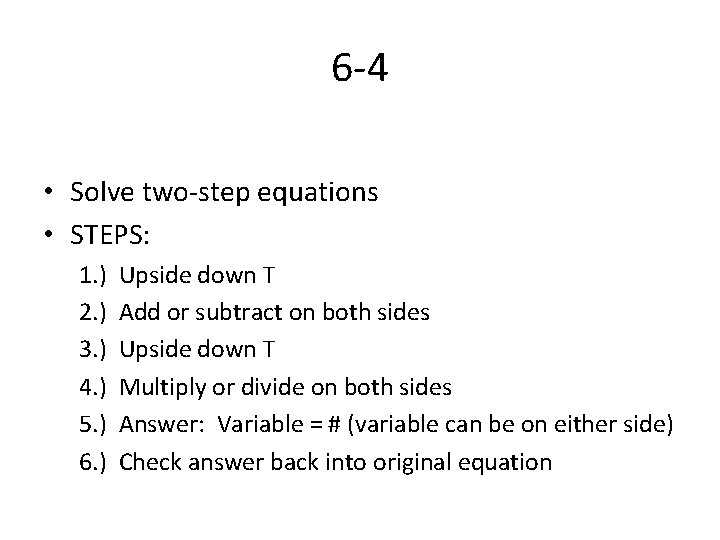6 -4 • Solve two-step equations • STEPS: 1. ) Upside down T 2. ) Add or subtract on both sides 3. ) Upside down T 4. ) Multiply or divide on both sides 5. ) Answer: Variable = # (variable can be on either side) 6. ) Check answer back into original equation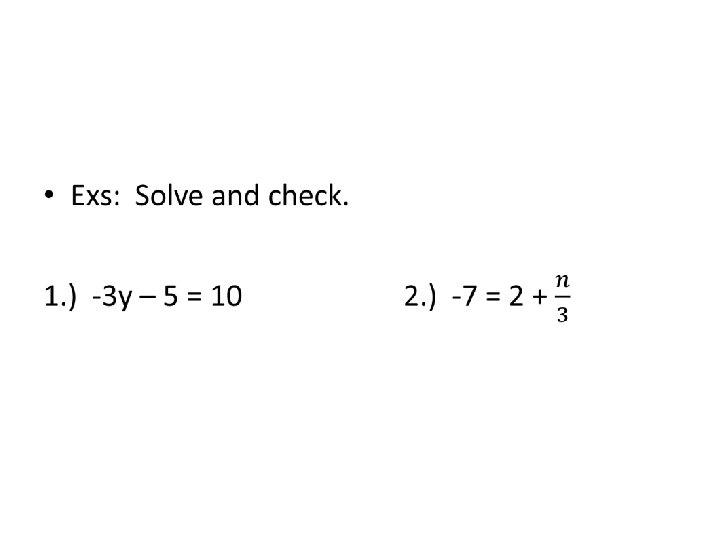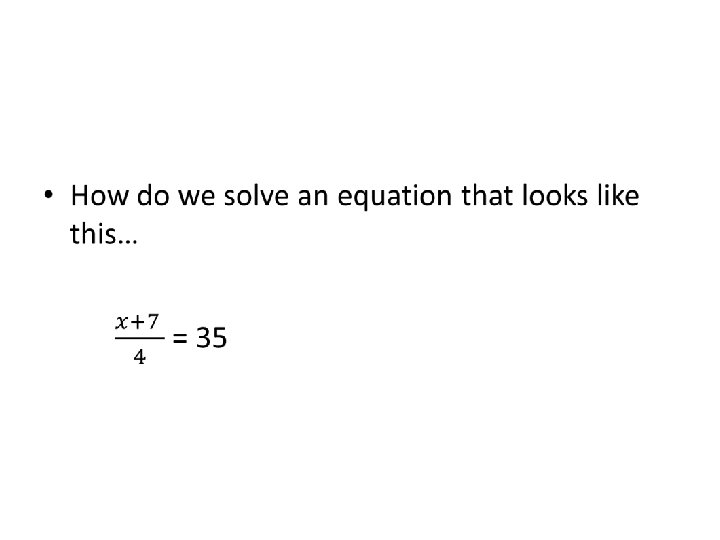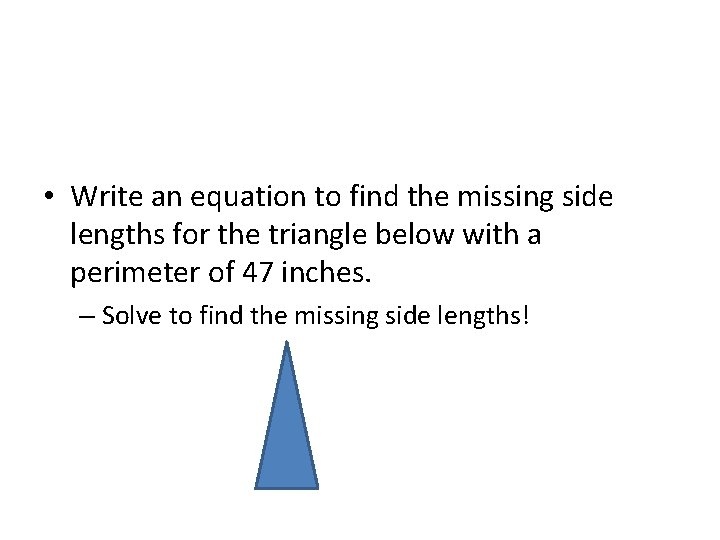• Write an equation to find the missing side lengths for the triangle below with a perimeter of 47 inches. – Solve to find the missing side lengths!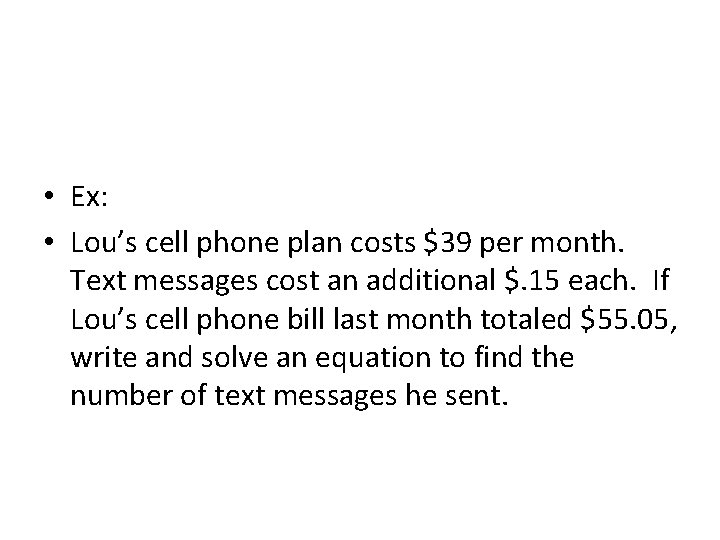• Ex: • Lou’s cell phone plan costs \$39 per month. Text messages cost an additional \$. 15 each. If Lou’s cell phone bill last month totaled \$55. 05, write and solve an equation to find the number of text messages he sent.6 -5 • Multi-step equations – Has parentheses OR – Has like terms on one side OR – Has 3 or more terms on one side • *Can only start solving when you have 2 or less terms on one side and you have combined any like terms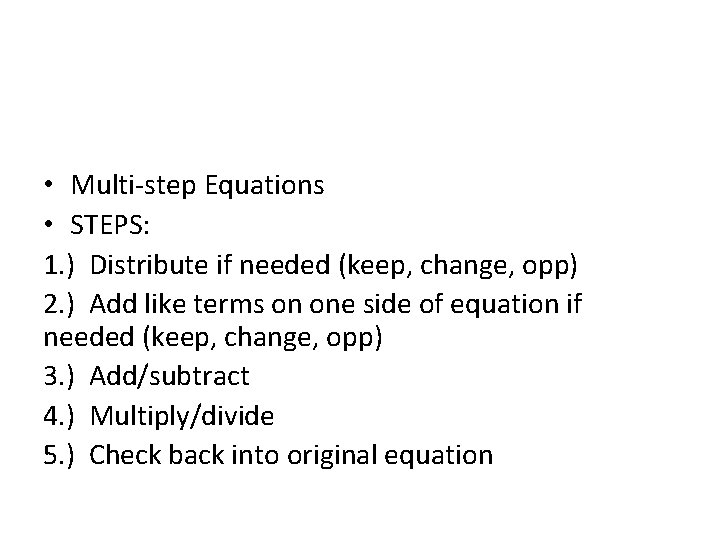• Multi-step Equations • STEPS: 1. ) Distribute if needed (keep, change, opp) 2. ) Add like terms on one side of equation if needed (keep, change, opp) 3. ) Add/subtract 4. ) Multiply/divide 5. ) Check back into original equation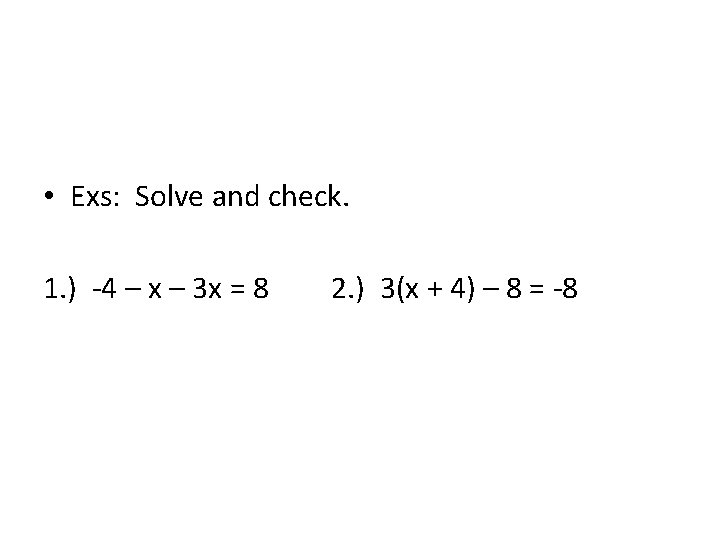• Exs: Solve and check. 1. ) -4 – x – 3 x = 8 2. ) 3(x + 4) – 8 = -8• Solve the following equations. Round to the nearest hundredth if necessary 1. ) -4(3 x + 5 + 8 x) = 7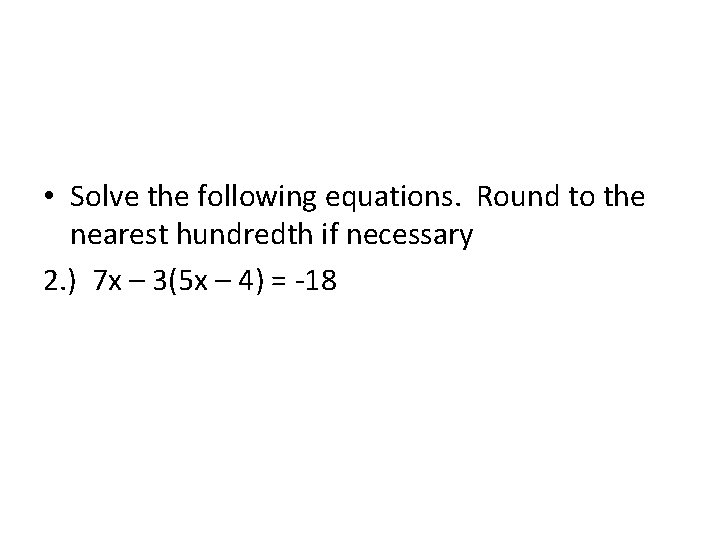• Solve the following equations. Round to the nearest hundredth if necessary 2. ) 7 x – 3(5 x – 4) = -18Writing Inequalities • Order: variable, inequality sign, number • Use key words in problem to figure out inequality sign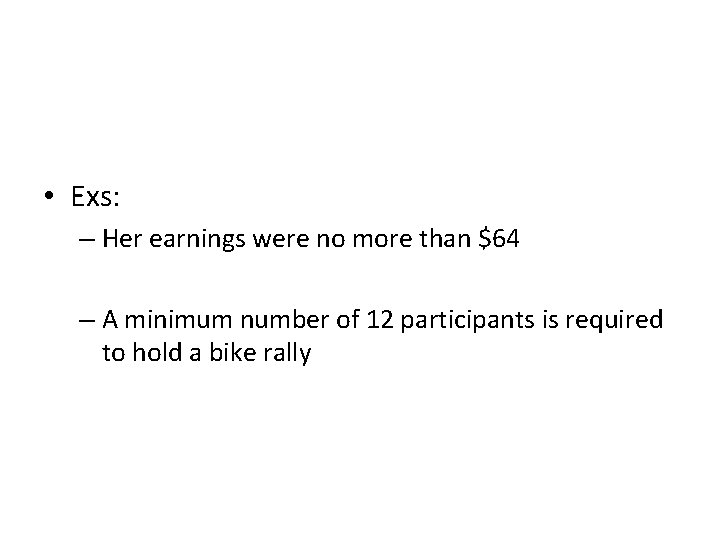• Exs: – Her earnings were no more than \$64 – A minimum number of 12 participants is required to hold a bike rally6 -6 • Solve one-step addition and subtraction inequalities *Solve inequalities the same way as equations • When adding or subtracting, bring inequality sign straight down for answer • Answer: variable, inequality sign, # – *Variable must be on the left!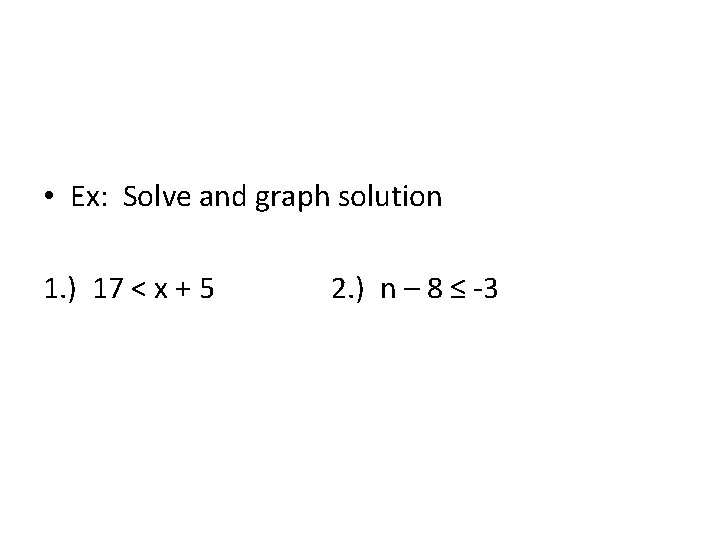• Ex: Solve and graph solution 1. ) 17 < x + 5 2. ) n – 8 ≤ -3• Ex: A hurricane has winds that are at least 74 miles per hour. Suppose a tropical storm has winds that are 42 miles per hour. Write and solve an inequality to find how much the winds must increase before the storm becomes a hurricane. Interpret the solution.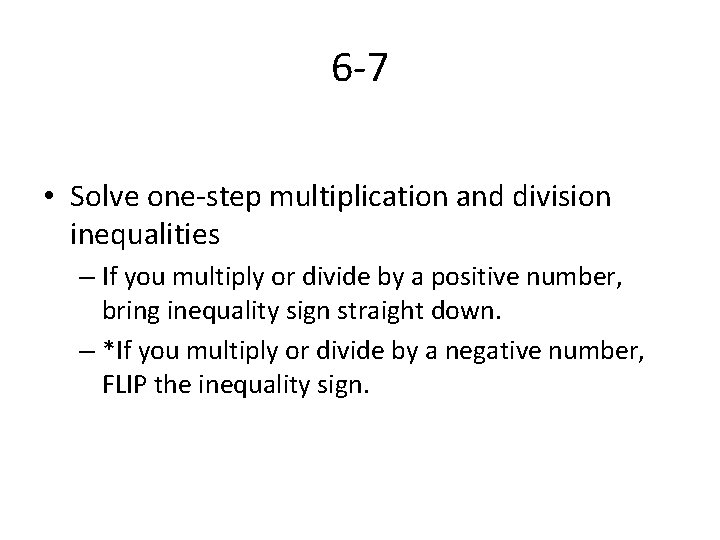6 -7 • Solve one-step multiplication and division inequalities – If you multiply or divide by a positive number, bring inequality sign straight down. – *If you multiply or divide by a negative number, FLIP the inequality sign.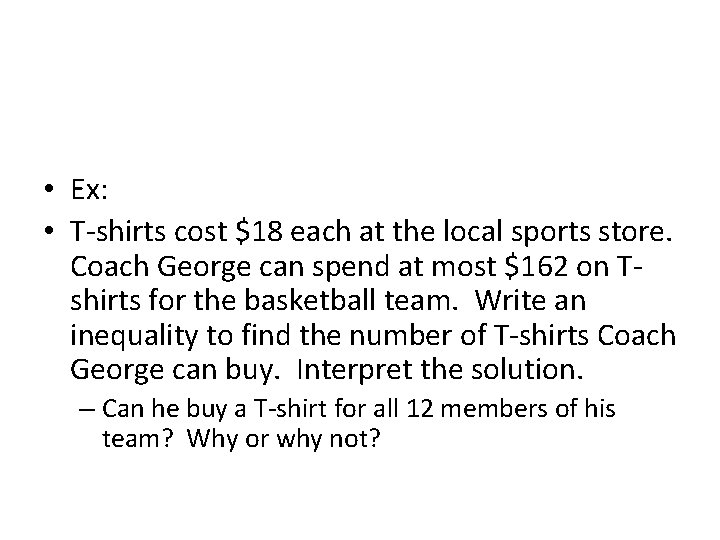• Ex: • T-shirts cost \$18 each at the local sports store. Coach George can spend at most \$162 on Tshirts for the basketball team. Write an inequality to find the number of T-shirts Coach George can buy. Interpret the solution. – Can he buy a T-shirt for all 12 members of his team? Why or why not?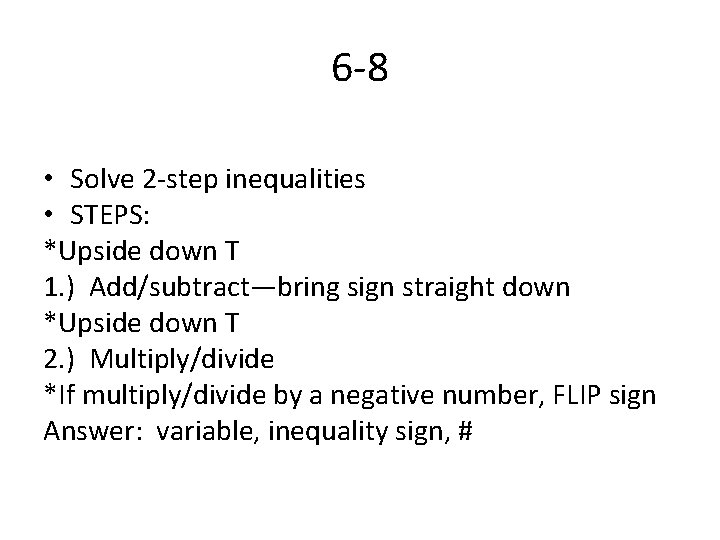6 -8 • Solve 2 -step inequalities • STEPS: *Upside down T 1. ) Add/subtract—bring sign straight down *Upside down T 2. ) Multiply/divide *If multiply/divide by a negative number, FLIP sign Answer: variable, inequality sign, #• Ex: Skate Land charges a \$50 flat fee for a birthday party rental and \$5 person. Joann has no more than \$100 to spend on the birthday party. Write an inequality to represent the situation. Interpret the solution. How many people can Joann invite to her party?Multi-step Inequalities • Solve multi-step inequalities – Has parentheses OR – Has like terms on one side OR – Has 3 or more terms on one side • *Can only start solving when you have combined any like terms and have 2 or less terms on variable side • *Make sure variable is on left or move it 1 st!• Solve multi-step inequalities • STEPS: 1. ) Distribute if needed (keep, change, opp) 2. ) Add like terms on one side of equation if needed (keep, change, opp) 3. ) Add/subtract—bring down sign 4. ) Multiply/divide *If you multiply/divide by negative #, FLIP SIGN 5. ) Graph solution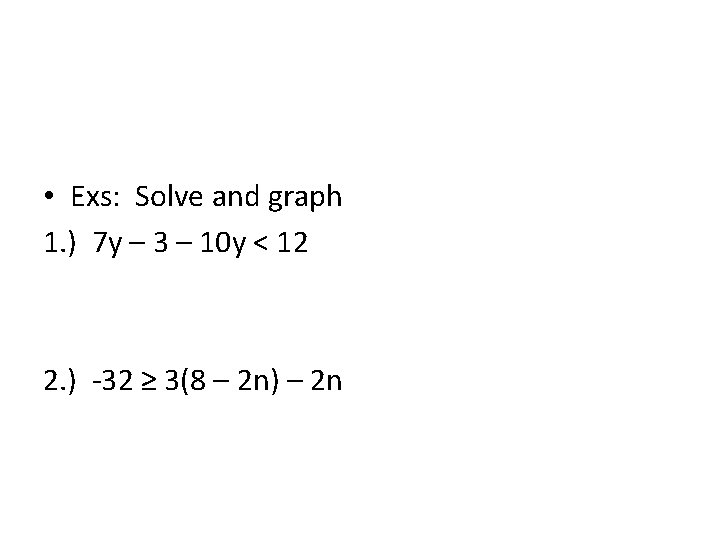• Exs: Solve and graph 1. ) 7 y – 3 – 10 y < 12 2. ) -32 ≥ 3(8 – 2 n) – 2 n